# Wavelet analysis

A wavelet is, roughly speaking, a (wave-like) function that is well localized in both time and frequency. A well-known example is the Mexican hat wavelet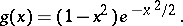(a1)

Another one is the Morlet wavelet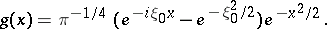(a2)

In wavelet analysis scaled and displaced copies of the basic waveletare used to analyze signals and images. The continuous wavelet transform of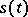is the function of two real variables,,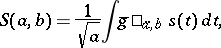(a3)

where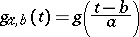and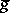is the complex conjugate of. In terms of the Fourier transform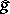ofone has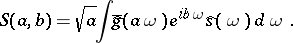(a4)

On the basic waveletone imposes the admissibility condition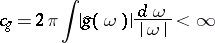(a5)

(which implies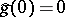, i.e.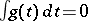, if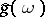is differentiable). Assuming (a5), there is the inversion formula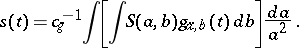(a6)

The wavelet transform is associated to the wavelet group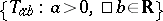,, and certain subgroups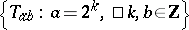in much the same way that the Fourier transform is associated with the groupsand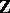. The early vigorous development of wavelet theory is mainly associated with the names of J. Morlet, A. Grosmann, Y. Meyer, and I. Daubechies, and their students and associates. One source of inspiration was the windowed Fourier analysis of D. Gabor, [a1].

An orthonormal wavelet basis is a basis of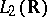of the formA non-differentiable example of such a basis is the Haar system. Orthonormal bases withof compact support and-times differentiable were constructed by Daubechies. These are called Daubechies bases. Higher differentiability, i.e. larger, for these bases requires larger support.

Wavelets seem particularly suitable to analyze and detect various properties of signals, functions and images, such as discontinuities and fractal structures. They have been termed a mathematical microscope. In addition, wavelets serve as a unifying concept linking many techniques and concepts that have arisen across a wide variety of fields; e.g. subband coding, coherent states and renormalization, Calderon–Zygmund operators, panel clustering in numerical analysis, multi-resolution analysis and pyramidal coding in image processing.